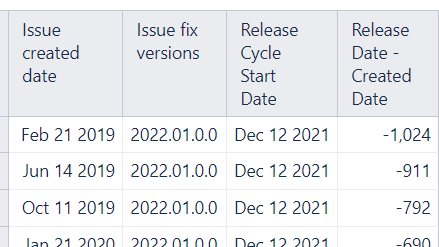I created 2 calculated measures, ‘Release Cycle Start Date’ and
The code for ‘Release Date - Created Date’ is:
CASE
WHEN CoalesceEmpty([Fix Version].[Version].getMemberNamesByKeys(
[Issue].CurrentHierarchyMember.get(‘Fix version IDs’),""))
MATCHES “2022.01.0.0”
THEN “Dec 12 2021”
WHEN CoalesceEmpty([Fix Version].[Version].getMemberNamesByKeys(
[Issue].CurrentHierarchyMember.get(‘Fix version IDs’),""))
MATCHES “2022.02.0.0”
THEN “Jan 23 2022”
ELSE “Jan 1 2030”
END

The purpose of this calculated measure is since our release values get inputed in the following format:
2022.01.0.0
2022.02.0.0
etc
I needed to convert these values to a date format so that I could compare them with another date format. So the calculated measure above basically assigns dates to these release values:
2022.01.0.0 > Dec 12 2021
2022.02.0.0 > Jan 23 2022

Then I created another calculated measure called ‘Release Date - Created Date’:
DateDiffDays([Measures].[Release Cycle Start Date],
[Measures].[Issue created date])

This calculate member simply subtracts the ‘Issue Created Date’ measure from the calculated measure that I created, ‘Release Cycle Start Date’. This part works. I’ll try to attach an image of this.So when you do this subtraction ‘Release Date - Created Date’, some release dates will be after the created date (which will give a positive value) and some release dates will be before the created date (which will give a negative value). What we’re trying to find out is how many Jira tickets were created before the Release Date and how many were created after the Release Date.

What I need to do now is create a report that looks something like this:

Here, ‘Release’ is same as ‘Fix Version’.

My issue is in creating the calculated measures for the two following fields:
Count Issues Created BEFORE Cycle Start Date
Count Issues Created AFTER Cycle Start Date

For ‘Count Issues Created BEFORE Cycle Start Date’, I’ve tried the various combinations but can get none of these attempts to work. Your help would be appreciated.

NonZero(Count(
Filter(Descendants([Fix Version].CurrentMember
,[Fix Version].[Version]),
[Measures].[Release Date - Created Date]) < 0
))

NonZero(Count(
Filter(Descendants([Issue].CurrentHierarchyMemberMember,[Issue].[Issue]),
[Measures].[Release Date - Created Date]) < 0
))

Count(
Filter(
Descendants([Issue].CurrentMember, [Issue].[Issue]),
DateDiffDays([Measures].[Release Cycle Start Date],
[Measures].[Issue created date]))) < 0

NonZero(Count(
Filter(Descendants([Issue].CurrentMember,[Issue].[Issue]),
[Measures].[Release Cycle Start Date] -
[Measures].[Issue created date]) < 0
)
))

Hi @alanv25,

You have done a great job with the calculations. The third option was very close - just a matter of brackets.
You have correctly found that you could use DateDiffDays for the date subtraction.

If you want to compare dates, you might use the function DateCompare.

Then you might refine the measure for “Created before cycle start Date” into the following expression.

``````NonZero(
--sum works a bit faster than count in MDX
Sum(
Filter(
DescendantsSet([Issue].CurrentHierarchyMember, [Issue].[Issue]),
--issue relates to the current report context - rows, columns, page filters
[Measures].[Issues created]>0
AND
--created before the relevant fix version start date
DateCompare([Issue].CurrentHierarchyMember.Get('Created at'),
[Measures].[Release Cycle Start Date])<0
),
--giving a number for the SUM
1
))
``````

Iterations through the Issues dimension tend to become slow for higher issue volumes, therefore you might address this task from a different angle.

Issues are already grouped on the Time dimension according to their creation date.
“Release Cycle Start Date” depends on the Fix version rather than the Issue.
The defined Fix version returns the issues related to this Fix version and the Fix version is already on report rows.

You might define a “Fix version cycle start date” based on the Fix version in the report context.

``````CASE
WHEN [Fix Version].CurrentHierarchyMember.Name MATCHES "2022.01.0.0"
THEN DateParse("Dec 12 2021")
WHEN [Fix Version].CurrentHierarchyMember.Name MATCHES "2022.02.0.0"
THEN DateParse("Jan 23 2022")
ELSE DateParse("Jan 1 2030")
END
``````

Although a lot of calculations would convert strings into datetime at need, defining the proper format is preferred, thus the DateParse function.

Retrieving the release cycle start date for the Fix Version allows using the PreviousPeriods function on the Time dimension to retrieve the issues created before the date.

Then the expression for the measure “Created before cycle start Date” might look like this.

``````NonZero(
Sum(
PreviousPeriods([Time].[Day].DateMember([Measures].[Fix version cycle start date])),
[Measures].[Issues created]))
``````

This set should be smaller and the calculation should work faster.

Regards,
Oskars / support@eazyBI.com

Thanks Oskar. Your answer is great! I got it working using the last 2 measures in your reply, but I could not find a formula that would get me “Created AFTER cycle start date”. I ended up getting the count of issues created after the cycle start date by subtracting the issues created before the cycle start date from the total number of issues.

1 Like